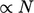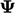## 5.23Summary of algorithms and solvers

• The ﬁnite volume method produces sparse matrices, Sec. 5.1 .
• Symmetric matrices have the same coeﬃcients across the diagonal and advection generally produces an asymmetric matrix.
• The matrix equations are solved using iterative methods that reduce a residual to a speciﬁed tolerance, Sec. 5.4 .
• Diagonal dominance is a suﬃcient condition for convergence, see Eq. (5.9 ) and Sec. 5.5 .
• Under-relaxation may be required to maintain diagonal dominance, Sec. 5.6 , in particular for steady-state solutions.

### Gauss-Seidel method

• Simple iterative method for solutions with cost, Sec. 5.2 .
• Eﬀective when the range of inﬂuence is short, i.e.in each cell is inﬂuenced by changes in close neighbouring cells.
• The symmetric variant and mesh renumbering may improve convergence, Sec. 5.8 .

• A “descent” method which solves a minimisation problem for cases with a longer range of inﬂuence, Sec. 5.14 .
• Solved iteratively, which requires preconditioning to make it eﬃcient, Sec. 5.16 .
• Symmetric matrix: PCG with DIC preconditioning.
• Asymmetric matrix: PBiCGStab with DILU preconditioning.

### Geometric-algebraic multi-grid (GAMG)

• Well suited to equations with a long range of inﬂuence, in particular the pressure equation, Sec. 5.17 .
• Simple algebraic agglomeration is corrected by a scaling based on minimisation for Laplacian-dominated equations, Sec. 5.18 .

### Systems of equations

• Systems of equations are solved iteratively with solutions from one equation substituted into the next, Sec. 5.9 .
• Mass and momentum conservation are coupled by a pressure equation and momentum and ﬂux correctors, Sec. 5.10 .